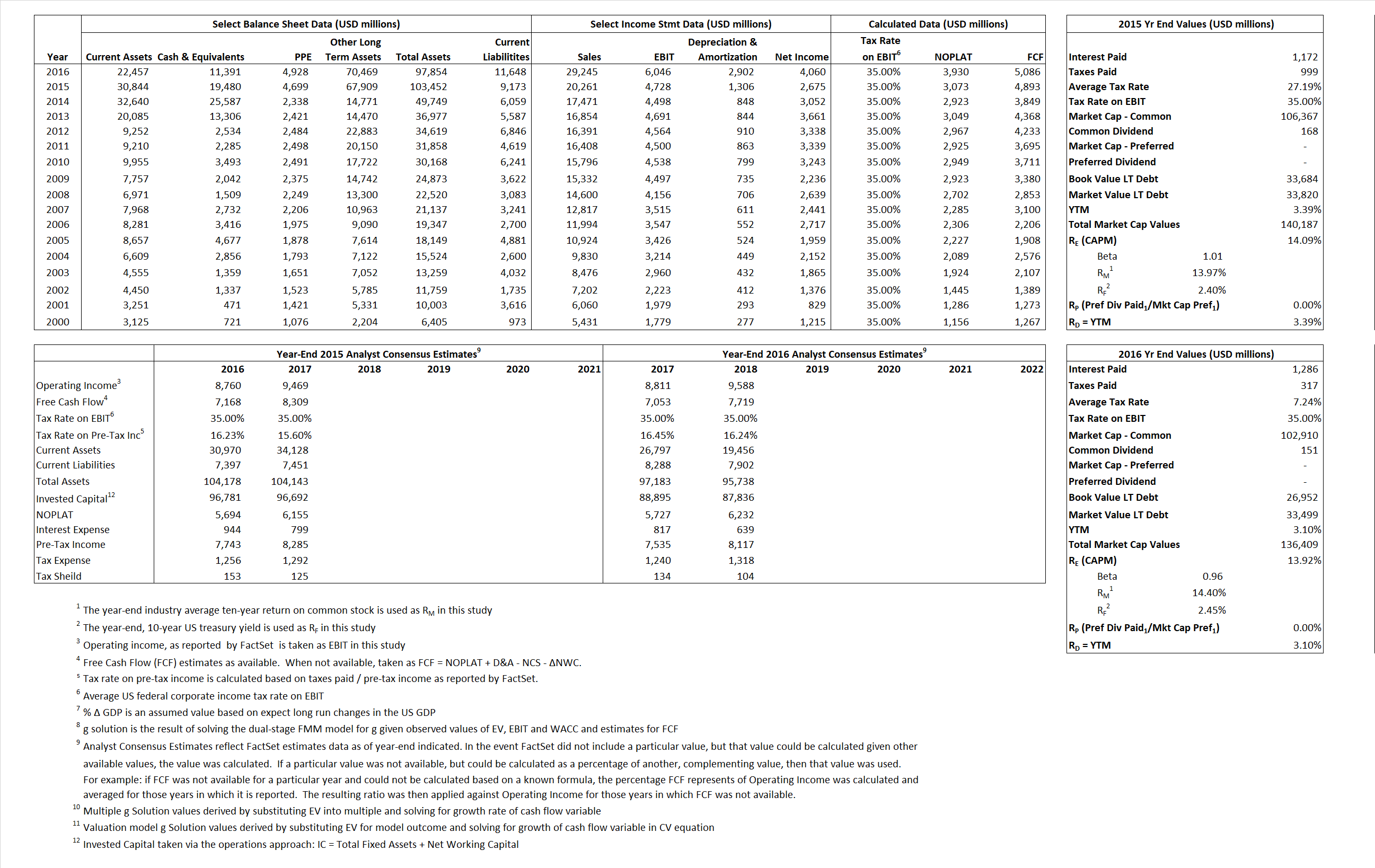# Medtronic Plc

## Analyst Listing

The following analysts provide coverage for the subject firm as of May 2016:

 Broker Analyst Analyst Email William Blair Ben Andrew bandrew@williamblair.com Piper Jaffray Brooks E. West brooks.e.west@pjc.com SunTrust Robinson Humphrey Bruce Nudell bruce.nudell@suntrust.com Leerink Partners Danielle Antalffy danielle.antalffy@leerink.com RBC Capital Markets Glenn Novarro glenn.novarro@rbccm.com BMO Capital Markets Joanne K. Wuensch joanne.wuensch@bmo.com Cowen & Company Joshua Jennings josh.jennings@cowen.com Deutsche Bank Research Kristen Stewart kristen.stewart@db.com Wells Fargo Securities Larry Biegelsen lawrence.biegelsen@wellsfargo.com Credit Suisse Matthew Keeler matthew.keeler@credit-suisse.com Needham Mike Matson mmatson@needhamco.com Jefferies Raj Denhoy rdenhoy@jefferies.com Stifel Nicolaus Rick Wise wiser@stifel.com BTIG Sean Lavin slavin@btig.com Oppenheimer Steven Lichtman steven.lichtman@opco.com Wedbush Securities Tao Levy tao.levy@wedbush.com Evercore ISI Vijay Kumar vijay.kumar@evercoreisi.com

## Primary Input Data## Derived Input Data

### Equational Form

Net Operating Profit Less Adjusted Taxes NOPLAT 3,073  3,930$NOPLAT\, =\, EBIT\, x\, (1 \,-\, Avg \,\,Tax\,\, Rate\,\, on\,\, EBIT)$
Free Cash Flow FCF 4,893 5,086$FCF\,=NOPLAT\,+\,Non-Cash\,Expenses-\Delta NWC\,-\,NCS$
Tax Shield TS 319 93$TS\,=\,Interest\,\,Paid\,\,x\,\, Avg \,\,Tax\,\,Rate\,\, on\,\, Pre-Tax\,\, Income$
Invested Capital IC 94,279 86,206$IC\,=\,Fixed\,\,Operating\,\,Assets\,\,+\,\,Net\,\, Working\,\, Capital$
Return on Invested Capital ROIC 3.26% 4.56%$ROIC\,=\,\frac { NOPLAT }{ IC }$
Net Investment NetInv  51,896 (5,171)$NetInv\,=\,{ {IC}_{1}}-{{IC}_{0}}+Depreciation$
Investment Rate IR 1,688.66%  -131.59%$IR\,=\,\frac {NetInv}{NOPLAT}$
Weighted Average Cost of Capital
WACCMarket  11.28% 11.21%$WACC\,=\,\frac { E }{ V } { R }_{ E }\,+\,\frac { P }{ V } { R }_{ P }\,+\,\frac { D }{ V } { R }_{ D }\left( 1- Avg\,\, Tax\,\,Rate\,\,on\,\,Pre-Tax\,\,Income \right)$
WACCBook     7.72%   7.37%
Enterprise value
EVMarket  120,707 125,018$EV\,=\,Market\,\,Cap\,\,Equity\,+\,\,Long\,\,Term\,\,Debt\,-\,Cash$
EVBook    128,660   118,471
Long-Run Growth
g = IR x ROIC
55.04%   -6.00% Long-run growth rates of the income variable are used in the Continuing Value portion of the valuation models.
g = %$\Delta$ GDP    2.50%   2.50%
Margin from Operations M    23.34%   20.67%$M\,\,=\,\,\frac{EBIT}{SALES}$
Depreciation/Amortization Rate D    21.64%   32.43%$D\,\,=\,\,\frac{D+A}{EBITDA}$

## Valuation Multiple Outcomes

The outcomes presented in this study are the result of original input data, derived data, and synthesized inputs.

### model g solution

12/31/2015 12/31/2016 12/31/2015 12/31/2016 12/31/2015 12/31/2016

EV/SALES$\frac {EV}{Sales} \,= \,\frac{ROIC\, -\, g}{ROIC\,(WACC\,-\,g)}\,(1\,-\,T)\,(M)$

5.96  4.27  39.91%  25.98%  30.72%  19.59%

EV/EBITDA$\frac {EV}{EBITDA} \,= \,\frac{ROIC\, -\, g}{ROIC\,(WACC\,-\,g)}\,(1\,-\,T)\,(1\,-\,D)$

20.00 13.97 39.91% 25.98%  30.72% 19.59%

EV/NOPLAT$\frac {EV}{NOPLAT} \,= \,\frac{ROIC\, -\, g}{ROIC\,(WACC\,-\,g)}$

39.28 31.81 39.91% 25.98%  30.72% 19.59%

EV/FCFOPS$\frac {EV}{FCF_{OPS}} \,= \,\frac{ROIC\, -\, g}{ROIC\,(WACC\,-\,g)}\,(1\,-\,T)$

24.67 24.58 39.91% 25.98%  30.72%  19.59%

EV/EBIT$\frac {EV}{EBIT} \,= \,\frac{ROIC\, -\, g}{ROIC\,(WACC\,-\,g)}\,(1\,-\,T)$

35.53 20.68 39.91% 25.98%  30.72% 19.59%

EV/IC$\frac {EV}{IC} \,= \,\frac{ROIC\, -\, g}{WACC\,-\,g}$

1.28 1.45 39.91% 25.98%  30.72% 19.59%